Courses

# Test: Bending Stresses in Beams

## 10 Questions MCQ Test Strength of Materials (SOM) | Test: Bending Stresses in Beams

Description
This mock test of Test: Bending Stresses in Beams for Mechanical Engineering helps you for every Mechanical Engineering entrance exam. This contains 10 Multiple Choice Questions for Mechanical Engineering Test: Bending Stresses in Beams (mcq) to study with solutions a complete question bank. The solved questions answers in this Test: Bending Stresses in Beams quiz give you a good mix of easy questions and tough questions. Mechanical Engineering students definitely take this Test: Bending Stresses in Beams exercise for a better result in the exam. You can find other Test: Bending Stresses in Beams extra questions, long questions & short questions for Mechanical Engineering on EduRev as well by searching above.
QUESTION: 1

### A 0.2 mm thick tape goes over a frictionless pulley of 25 mm diameter. If E of the material is 100 GPa, then the maximum stress induced in the tape is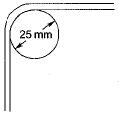Solution: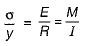(Bending formula)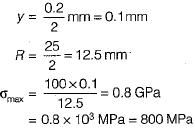QUESTION: 2

### A cantilever beam of rectangular cross-section is 1 m deep and 0.6 m thick. If the beam were to be 0.6 m deep and 1 m thick then the beam would

Solution: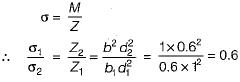QUESTION: 3

### The product El is known as

Solution:
QUESTION: 4

The assumption “the plane section before bending remains plane after bending” made in the theory of bending implies:

Solution:
QUESTION: 5

At the neutral axis of simply beam, there is

Solution:

Bending stress,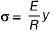where y is the distance of any fibre from the neutral axis.
For the neutral axis, y = 0, ∴ σ = 0

QUESTION: 6

For a beam of uniform strength, if its depth is kept constant, then its width will vary in proportion to

Solution: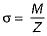for beam of uniform strength, σ = constant at any section
so, M α Z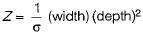∴ M α width

QUESTION: 7

When a rectangular beam is loaded longitudinal, shear develops on

Solution:
QUESTION: 8

A beam is said to be of uniform strength, if

Solution:
QUESTION: 9

A rod of length L and diameter D is subjected to a tensile load P. Which of the following is sufficient to calculate the resulting change in diameter?

Solution:
QUESTION: 10

Beams of uniform strength so vary in section that the

Solution:

Unit stress means stress at any section.